Mobile QR Code1. (School of Electrical Engineering, KAIST, Daejeon 34141, Korea)
2. (Department of Information and Communication Engineering, DGIST, Daegu 42988, Korea)

Neural recording, biomedical device, low-power, low-noise amplifier, noise efficiency factor, current-reuse, current-controlled pseudoresistor, fully differential front-end

## I. INTRODUCTION

According to the statistics released by the Christopher & Dana Reeve Foundation, approximately 5 million people, or about 1.7 % of the U. S. population, are paralyzed. It is also reported that the leading cause of paralysis is the stroke with 34 %, followed by the spinal cord injury with 27 %, multiple sclerosis with 19 %, cerebral palsy with 7 %, and the rest with 12 %. Around the world, there have been a number of attempts to restore the motor function of people suffering from the paralysis caused by the stroke, spinal cord injury, and so on. Among the rehabilitation treatments for paralysis patients, many studies have been carried out to read the brain signals of the paralysis patient and restore the motor function using external devices[2-5].

Monitoring neural signals is essential in the field of neuroprosthesis for restoring the motor function. Recorded neural signals are used to interpret the patient’s intention through signal processing. The external device is then controlled by the outcome of signal processing to create the motor function as intended by the patient. Fig. 1 illustrates the conceptual diagrams of the neuromotor prosthesis. Fig. 1(a) shows the system using an external robotic arm, which generates arm movements according to the patient’s intention. On the other hand, Fig. 1(b) depicts the system consisting of the neural recording subsystem and the neural stimulation subsystem, which electrically stimulates the patient’s arm muscles so that the arm can be moved according to the recorded and interpreted brain signals.

Fig. 1. Conceptual diagrams of restoring the motor function by (a) controlling an external robotic device, and (b) stimulating the patient’s arm muscles electrically.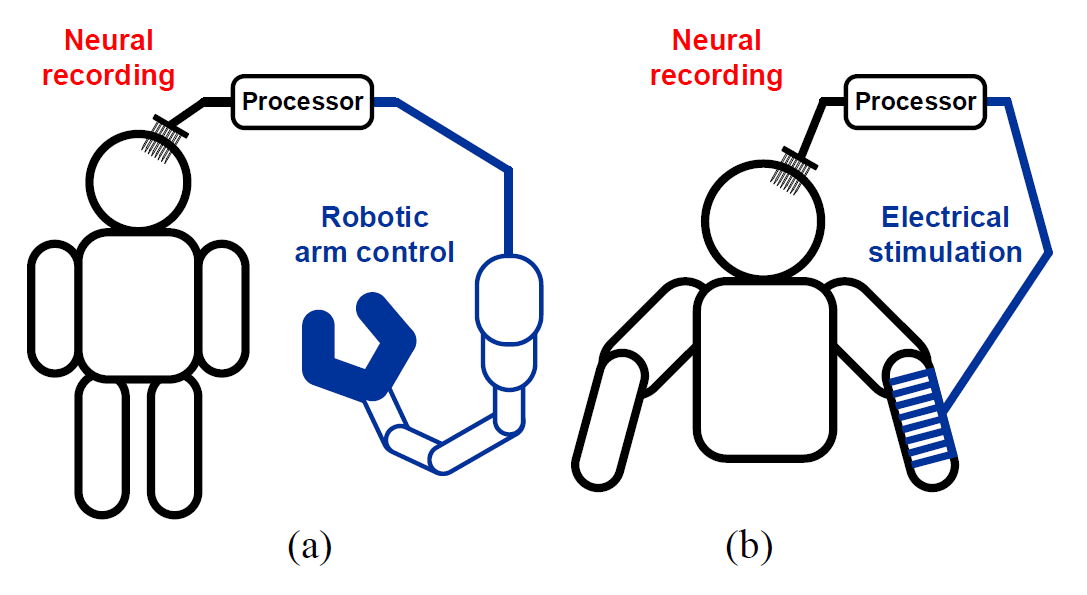Especially, for neuroprosthesis, implantable neural recording is preferred because of the high signal-to-noise characteristics of the acquired brain signals. Through the implantable recording, high-resolution neural signals can be obtained compared to the case of noninvasive neural recording. In addition, due to the larger signal amplitude, the requirement on low-noise performance for the implantable recording system can be relieved. However, the power consumption should be minimized to prevent cell necrosis caused by heat generation as well as to increase the battery lifetime of the implantable device.

To acquire the necessary information from the brain signals, mainly two kinds of extracellular signals shown in Fig. 2(a) need to be recorded in the implantable system.

Fig. 2. Electrical characteristics of (a) brain signals, (b) disturbances.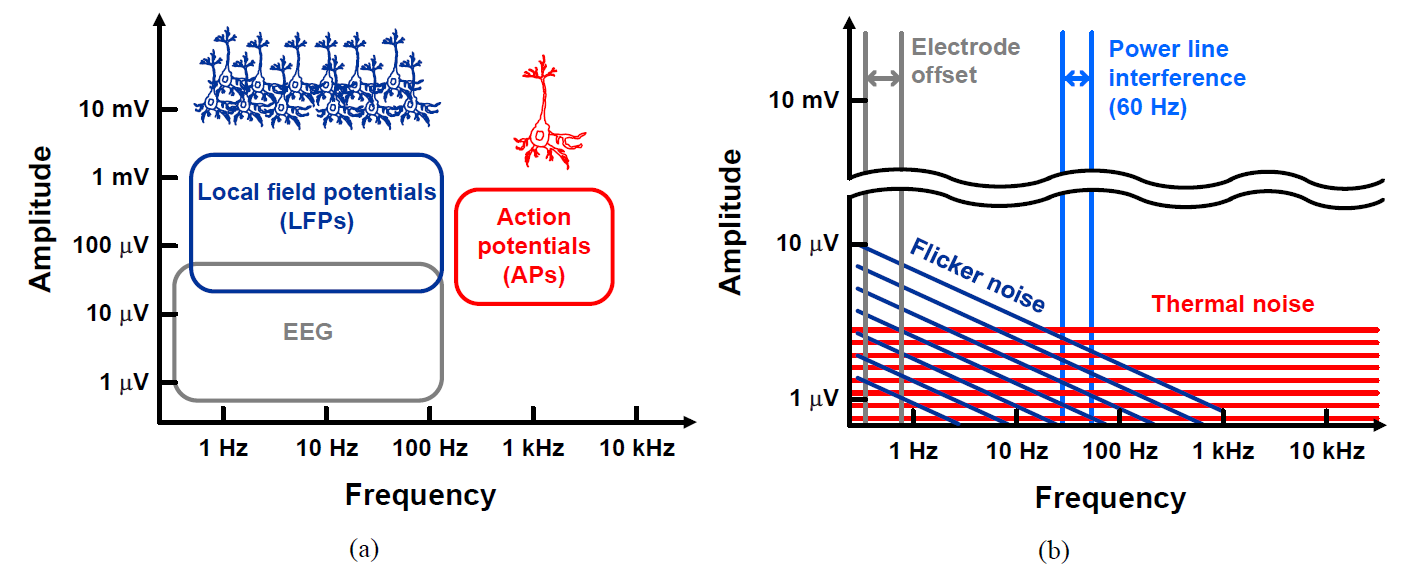When measuring the extracellular potential using the microelectrode array, several factors shown in Fig. 2(b) that can contaminate neural signals must be considered in the front-end IC design. DC offsets appear at the tissueelectrode interface due to electrochemical interaction. To avoid the electrical saturation of the front-end IC, the DC offsets must be suppressed. Two kinds of noise which are induced from the circuit are added to input neural signals. The flicker noise becomes dominant in the low-frequency region and affects the recording of LFPs. Since the spectrum of APs resides in the higher frequencies than LFPs, the recording of APs is less affected by the flicker noise, but more by the thermal noise. This is why, the front-end IC needs to be designed to have low-noise performance for observing clear extracellular potentials. Power line interferences are also coupled to the front-end IC. Even if the interferences appear as a common-mode signal at the frequency of 60 Hz, it is converted into the differential signal due to the mismatch between tissue-electrode impedances and circuit input impedances.

This paper presents a fully differential neural recording front-end IC for implantable microsystems. Each analog front-end (AFE) consists of a low-noise amplifier (LNA), a variable gain amplifier (VGA), and a buffer. The output signal of the AFE is digitized through a successive approximation register analog-to-digital converter (SAR ADC). The AFE is designed to have the high-pass cutoff frequency of sub-1 Hz using the currentcontrolled pseudoresistor. The AFE achieves the bandwidth of 10 kHz with programmable gains of 61.9 dB, 64.4 dB, 67.9 dB, and 73.9 dB. The inputreferred noise of 2.88$\mu$Vrms is measured when integrated from 0.5 Hz to 10 kHz, resulting in the noise efficiency factor (NEF) of 2.38 and the power efficiency factor (NEF2VDD) of 5.67. Each AFE occupies the area of 0.11 mm2/Channel and consumes the power of 4.6 $\mu$W/Channel under 1-V supply voltage. The front-end IC is implemented using a standard 1P6M 0.18-$\mu$m CMOS process.

This paper is organized as follows. The overall system architecture is described in Section II. The design details of each block are described in Section III, followed by the measurement results presented in Section IV. Finally, Section V concludes this paper.

## II. PROPOSED ARCHITECTURE

The overall system architecture is presented in Fig. 3. Eight AFEs share a single SAR ADC through the analog multiplexer. Each AFE consists of the LNA, VGA, and buffer. The AFE is implemented as a fully differential structure to be robust against common-mode interferences. The DC electrode offsets are suppressed by designing the AFE as an AC-coupled structure. Fig. 4(a) shows the schematic of the AC-coupled amplifier used in the LNA and VGA. To observe the low-frequency neural signals, the high-pass cutoff frequency of the neural amplifier needs to be low enough while eliminating the DC electrode offsets. Therefore, a high resistance is required in the feedback path as shown in Fig. 4(a). The high-pass cutoff frequency fHPF and voltage gain are expressed as 1/($2 \pi R C _{2}$) and $C _{1}$/$C _{2}$, respectively (Fig. 4(b)). To obtain low fHPF, a large value is required for the time constant formed by the resistance and capacitance in the feedback path of the LNA. However, to obtain sufficient voltage gain, the value of $C _{1}$ cannot be large at all, meaning that the value of R has to be extremely large.

Fig. 3. Block diagram of the fully differential implantable neural recording front-end IC.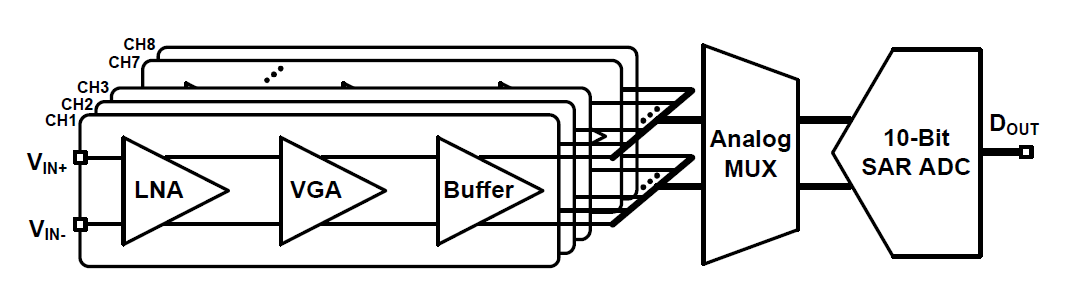Fig. 4. (a) Circuit schematic, (b) gain-frequency characteristic of the AC-coupled amplifier.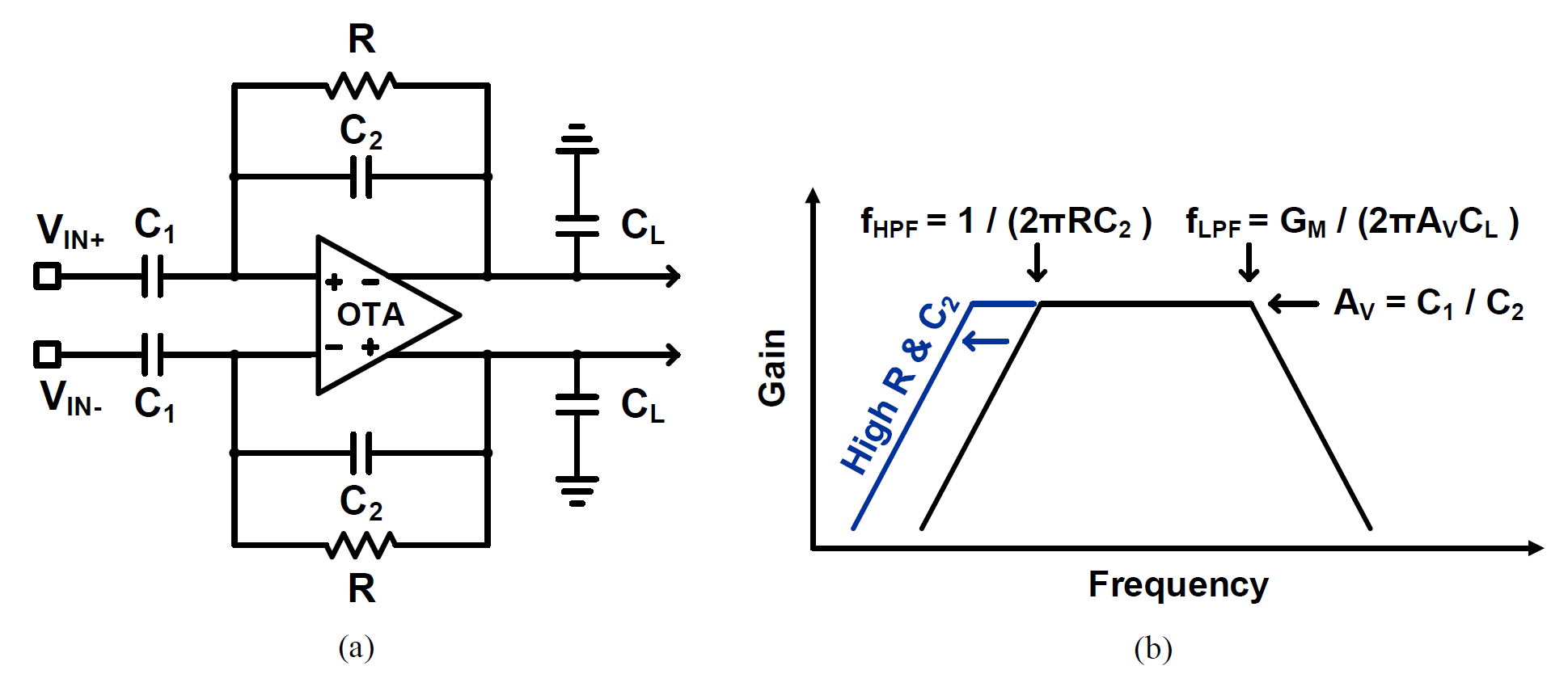To realize the large value of R and achieve low fHPF while occupying the small area, a pseudoresistor is conventionally employed as a diode-connected MOSFET or a series combination of them (Fig. 5).

However, the pseudoresistor implemented using diodeconnected MOSFETs is sensitive to process variations, and hence the high value of $R$ resulting in low $f_{HPF}$ is not guaranteed easily[7,9]. To overcome this issue, a voltage-controlled pseudoresistor is used by tuning the gate voltage of the MOSFET. Fig. 6 shows three such kinds of pseudoresistors controlled by tuning their gate voltages[10-12]. The voltage-controlled pseudoresistor can achieve a high resistance but requires extremely fine voltage regulation, which is impractical. Another robust way to implement a high resistance is to use a currentcontrolled pseudoresistor, which maintains the voltage difference between its gate and source terminals by mirroring the reference current source.

Fig. 6. Schematic of the voltage-controlled pseudoresistor.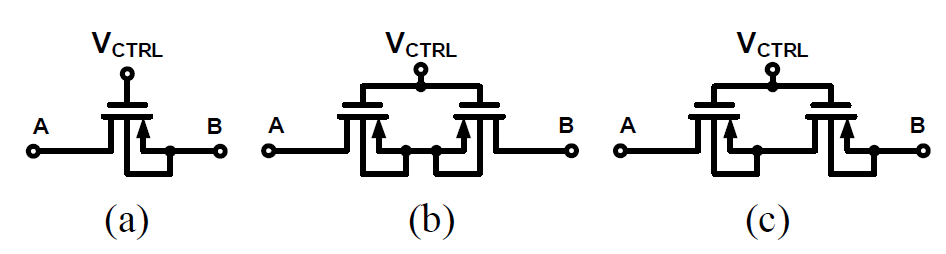In this paper, two types of modified current-controlled pseudoresistor designs are proposed, and their performances are compared with those of the simple diode-connected pseudoresistor and the voltagecontrolled pseudoresistor. Fig. 7 shows the schematic diagrams of a bias generator (BG), a type-1 currentcontrolled pseudoresistor (CCPR-1), and a type-2 current-controlled pseudoresistor (CCPR-2).

As shown in Fig. 7, the BG copies the reference current $I_{REF}$ to the branch formed by $M _{4}$ and $M _{5}$. The bias voltage, $V_{BIAS}$ generated from the BG, is connected to the gate terminals of $M _{R1}$ and $M _{R2}$ in CCPR-1/CCPR-2. The copied current flows through $M _{R1}$ and $M _{R3}$. The current flowing through $M _{R3}$ maintains a constant voltage drop between its gate and source terminals ($V_{GS}$). Then, the $V_{GS}$ of $M _{R5}$ follows the $V_{GS}$ of $M _{R3}$. Similarly, the copied current flows through $M _{R2}$ and $M _{R4}$. Then, the $V_{GS}$ of $M _{R6}$ follows the $V_{GS}$ of $M _{R4}$. The $M _{R5}$ and $M _{R6}$ operate as a current-controlled pseudoresistor. In CCPR-1, the gate terminals of $M _{R3}$ and $M _{R4}$ sense the node voltages at A and B, respectively. In CCPR-2, the gate terminals of $M _{R3}$ and $M _{R4}$ share the sensing point to reduce the mismatch between the $V_{GS}$ values of $M _{R3}$ and $M _{R4}$.

Fig. 7. Schematic of the current-controlled pseudoresistor.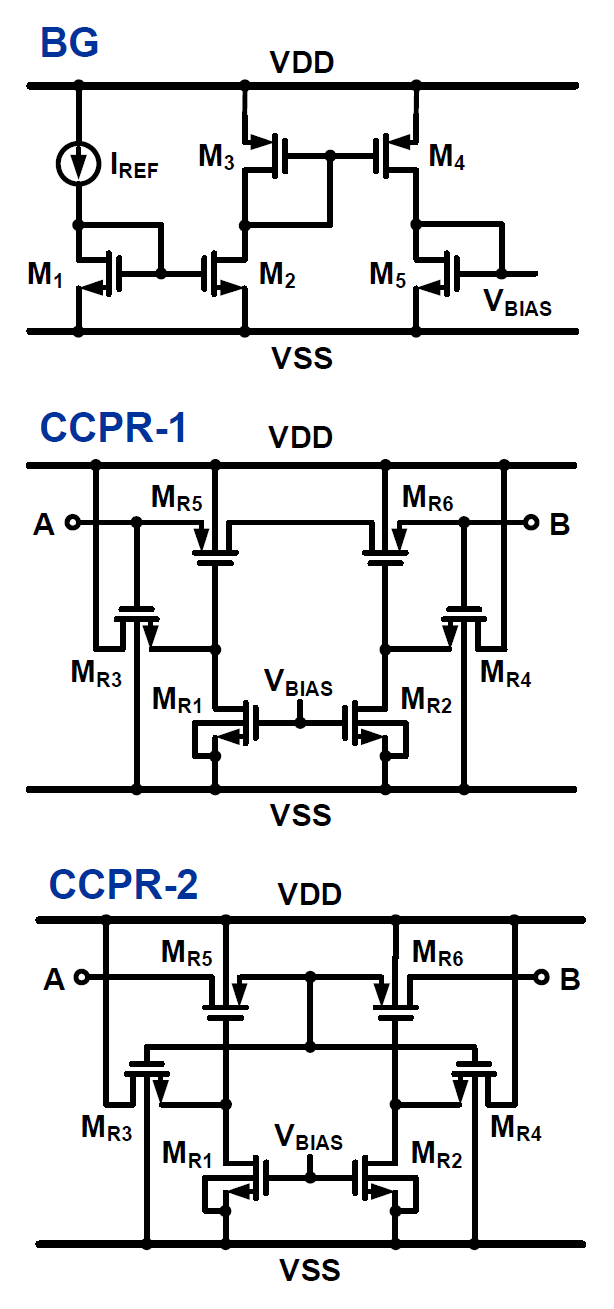Fig. 8 shows the schematic of the AFE consisting of the LNA, VGA, and buffer. The gain of the LNA is set to 40 dB by using $C_{1}$ and $C_{2}$ of 10 pF and 100 fF, respectively. The system gain is programmed through the VGA, which controls its gain from $C_{3}$/4$C_{U}$ to $C_{3}$/$C_{U}$ using the switches, $G_{1}$, $G_{2}$, and $G_{3}$. $C_{3}$ and $C_{U}$ are set to 5 pF and 100 fF, respectively. The pseudoresistor $R_{pseudo}$ is connected in parallel with $C_{2}$ and $C_{4}$ to set the input DC bias point of the operational transconductance amplifier (OTA) as well as to achieve low $f_{HPF}$. The ADC driving buffer is designed to have a rail-to-rail structure. The AFE performs fast DC bias settling and removes accumulated charges when the reset signal $V_{RST}$ is high. The size of each AFE is 82 μm × 1,260 μm as shown in Fig. 8.

Fig. 8. Schematic of the analog front-end consisting of the low-noise amplifier, variable gain amplifier, and buffer (top) and layout of the analog front-end occupying the area of 82 μm × 1,260 μm (bottom).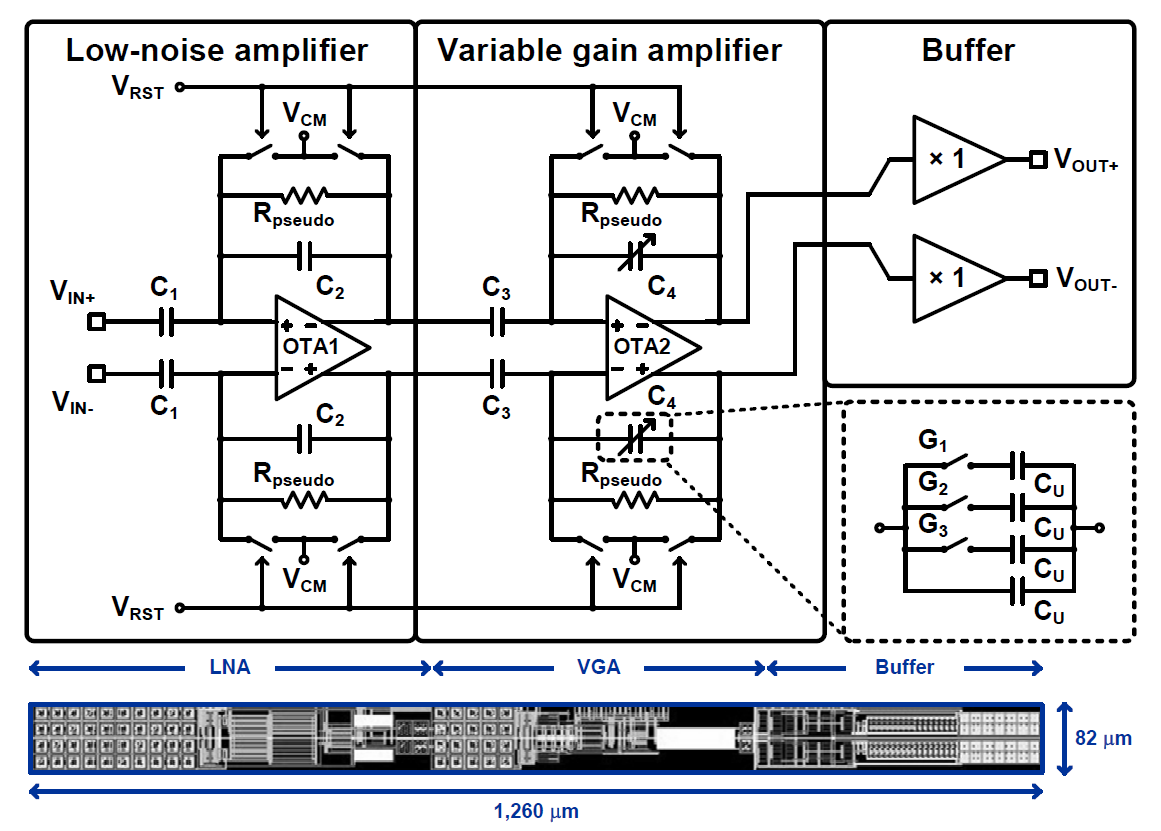## III. CIRCUIT DESIGN

The LNA has a fully differential structure and employs the current-reuse technique to improve the current efficiency. Fig. 9 shows the schematic of the OTA1 used in the LNA. In the input stage of the OTA1, $M _{1}$–$M _{4}$ construct the current-reuse scheme, which boosts the transconductance of the OTA1 for the given current consumption and reduce the input-referred noise[9,11,14-17]. For the same reason, the input stage operates in the weak inversion to increase the transconductance under the given bias current. The flicker noise is reduced by making the size of $M _{1}$–$M _{4}$ large. The size of $M _{1}$ and $M _{2}$ is set to 200 μm / 1 μm, and the size of $M _{3}$ and $M _{4}$ is set to 300 μm / 2 μm. $M _{5}$ and $M _{6}$ provide a common-mode feedback (CMFB) for the input stage without additional power consumption[14-17]. The second stage is added after the input stage to increase the open-loop gain, which is designed to be 75 dB. The output DC bias point is set by the CMFB circuit, which consists of $M _{11}$–$M _{14}$. Output voltages of $V _{O+}$ and $V _{O–}$ are sensed using diode-connected MOSFETs. The sensed voltage is applied to the gate terminal of $M _{11}$. The output of the CMFB circuit is fed back to the shared gate terminal of $M _{9}$ and $M _{10}$ in the second stage. Then, the DC bias level of $V_{O+}$ and $V_{O–}$ follows the common-mode voltage $V_{CM}$. The frequency compensation is performed using $R_{Z}$ and $C_{M}$. The phase margin of the OTA1 including the CMFB circuit is designed to be more than 45° with the load capacitance of 10 pF. The total current consumption of the OTA1 is 950 nA. Since the input stage mostly determines the noise performance, the bias current of 730 nA is used for the input stage. The second stage and CMFB circuit consume 110 nA and 110 nA, respectively. Unlike the fully differential current-reuse OTA design in  that employs two explicit CMFB circuits for both the first and second stages, the OTA design used here employs only one explicit CMFB circuit, which is for the second stage. Hence, the circuit design is simplified and the power consumed by the CMFB circuit for the first stage can be saved. The closed-loop gain is set by the ratio of $C_{1}$/$C_{2}$. The DC bias point of the OTA1 inputs follows that of the output DC voltages due to the DC feedback path formed by $R_{pseudo}$.

Fig. 9. Schematic of the OTA1 used in the low-noise amplifier.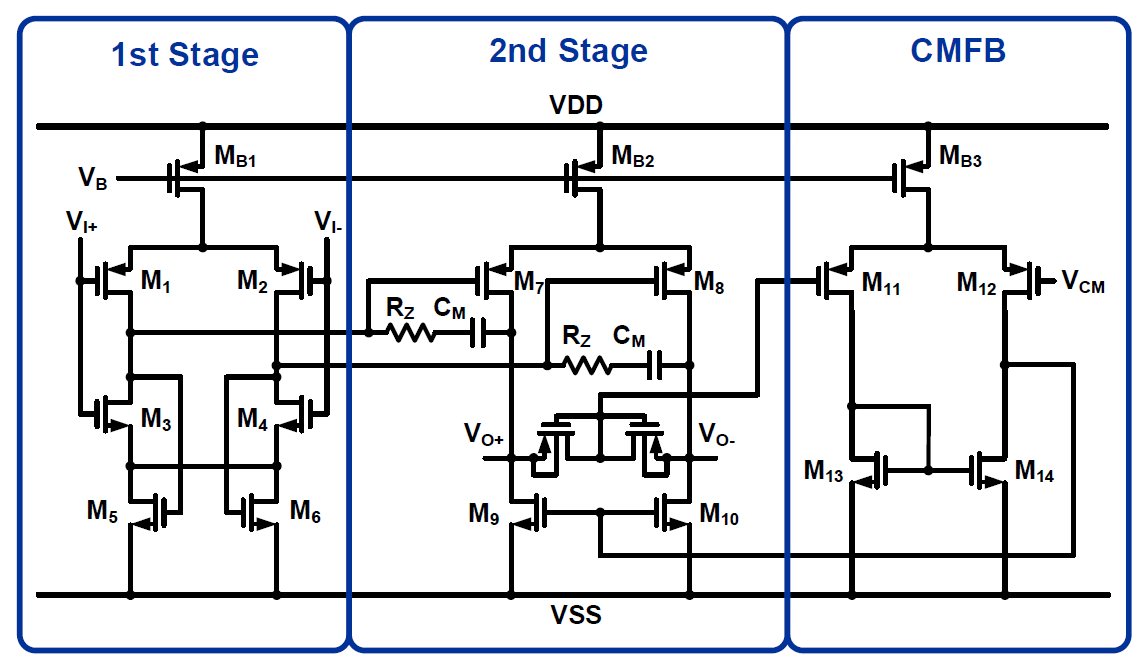Since the neural signals are first amplified by the LNA, the requirement on the noise performance of the VGA can be mitigated. Therefore, the current consumption of the OTA2 can be much reduced compared to that of OTA1. Fig. 10(a) shows the schematic of the OTA2 used in the VGA. The input stage is composed of a pair of PMOS transistors, $M _{1}$ and $M _{2}$. The outputs of $V_{O+}$ and $V_{O–}$ are sensed using diode-connected MOSFETs and transferred to the input of the CMFB circuit. The output voltage of the CMFB circuit is fed back to the gate terminals of $M _{3}$ and $M _{4}$. Then, the common-mode voltage of $V_{O+}$ and $V_{O–}$ is set to $V_{CM}$. The frequency compensation is implemented using $R_{Z}$ and $C_{M}$. The phase margin of OTA2 including CMFB circuit is designed to be more than 68° with the load capacitance of 1 pF. The open-loop gain is 100 dB. The OTA2 consumes the current of 350 nA including the CMFB circuit. The closed-loop gain can be varied from 21.9 dB to 33.9 dB.

Fig. 10(b) shows the schematic of the buffer that drives the capacitive DAC of the SAR ADC. It employs complementary input pairs composed of an NMOS pair and a PMOS pair to construct the rail-to-rail structure. The buffer is designed to achieve the phase margin of more than 58° with the load capacitance of 10 pF. The buffer consumes the power of 1.64 μW. In each AFE, the reset path is connected in parallel with $R_{pseudo}$ for fast settling of DC bias point and removal of accumulated charges when $V_{RST}$ is high.

Fig. 10. Schematic diagrams of (a) OTA2 used in the variable gain amplifier, (b) buffer.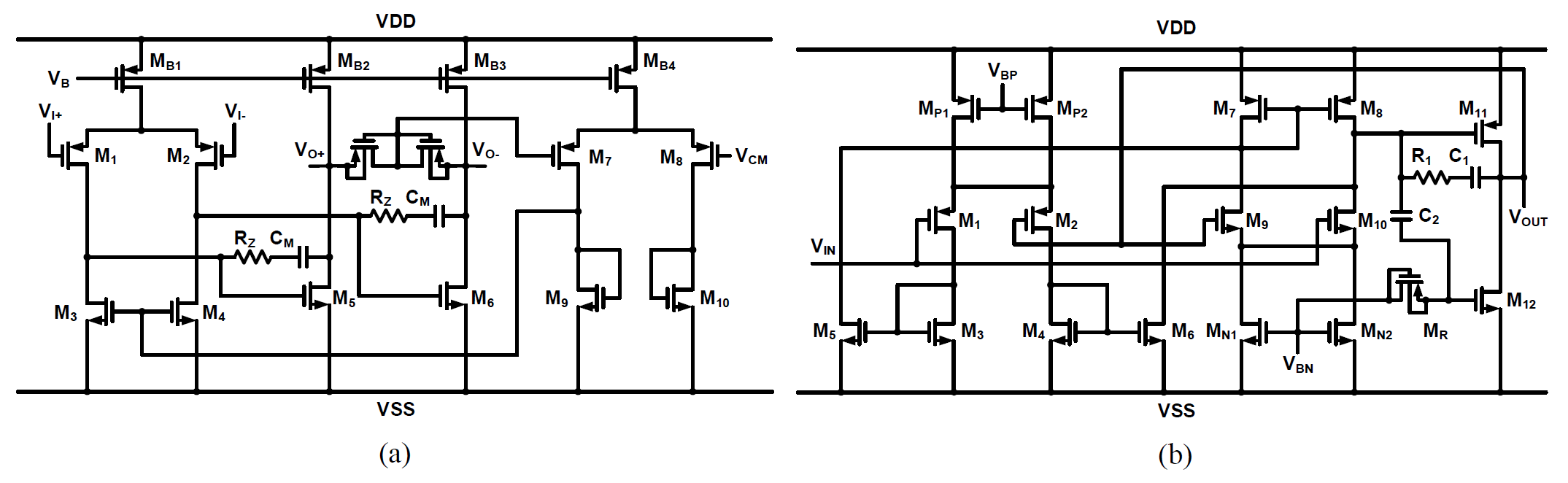## IV. MEASUREMENT RESULTS

The neural recording front-end IC has been fabricated using a standard 1P6M 0.18-μm CMOS process. The chip is measured with 1-V supply voltage. The measurement results present the high-pass cutoff frequency characteristics when the diode-connected pseudoresistor, voltage-controlled pseudoresistor, and current-controlled pseudoresistor are used. Fig. 11 shows the frequency response of the LNA with the diodeconnected pseudoresistor, exhibiting $f _{HPF}$ of 10 Hz. The frequency response with the voltage-controlled pseudoresistor is presented in Fig. 12, which is measured for the scheme shown in Fig. 6(a). The $f _{HPF}$ is controlled by tuning the gate voltage $V_{CTRL}$. The $f _{HPF}$ changes from 17 Hz to 1 Hz as $V_{CTRL}$ increases from 0.5 V to 0.8 V. Fig. 13 shows the frequency response with CCPR-1 and CCPR-2. As shown in Fig. 7, CCPR-1 and CCPR-2 are driven by copying the reference current $I_{REF}$, which is set to 5 μA. CCPR-1 and CCPR-2 create the $f _{HPF}$ of 1.2 Hz and 0.06 Hz, respectively. To compare the low-frequency output signals when using CCPR-1 and CCPR-2, the input signal of 2 μVpp at 0.5 Hz is applied to the LNAs adopting CCPR-1 and CCPR-2. The LNA with CCPR-1 shows the gain of 34 dB at 0.5 Hz, whereas the LNA with CCPR-2 shows the gain of 39 dB at 0.5 Hz as shown in Fig. 13 (bottom). As a result, the LFPs can be measured with minimal loss. Fig. 14 shows the output signals of the LNA when applying the input signals of 2 μVpp at 1 kHz, 2 kHz, and 4 kHz.

Fig. 11. Frequency response of the LNA using the conventional pseudoresistor.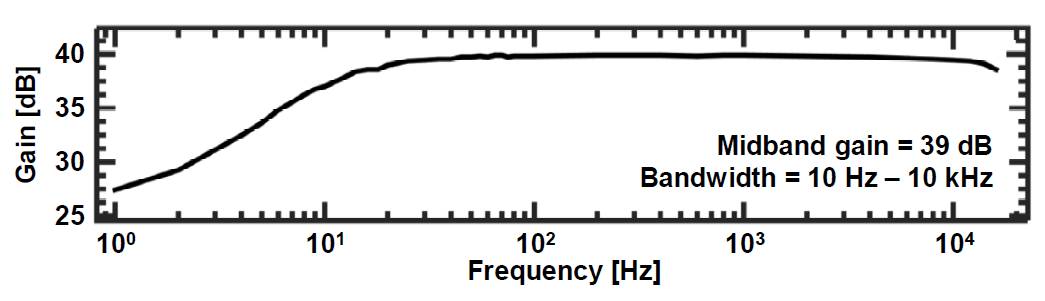Fig. 12. Frequency response of the LNA using the voltagecontrolled pseudoresistor shown in Fig. 6(a).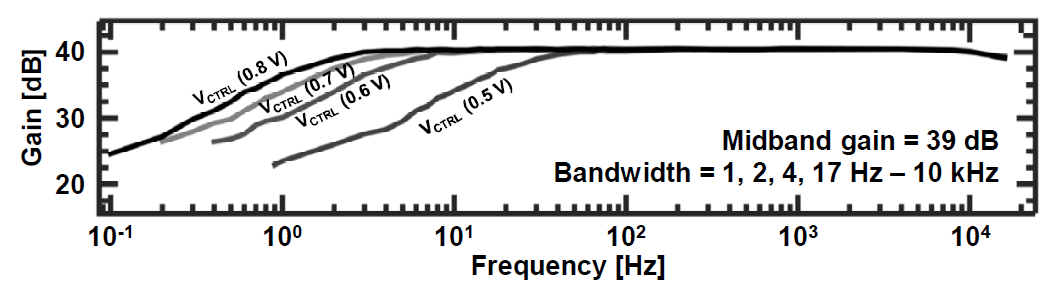Fig. 13. Frequency response of the LNA using the currentcontrolled pseudoresistor (top) and output signals when applying the input signal of 2 μVpp at 0.5 Hz (bottom).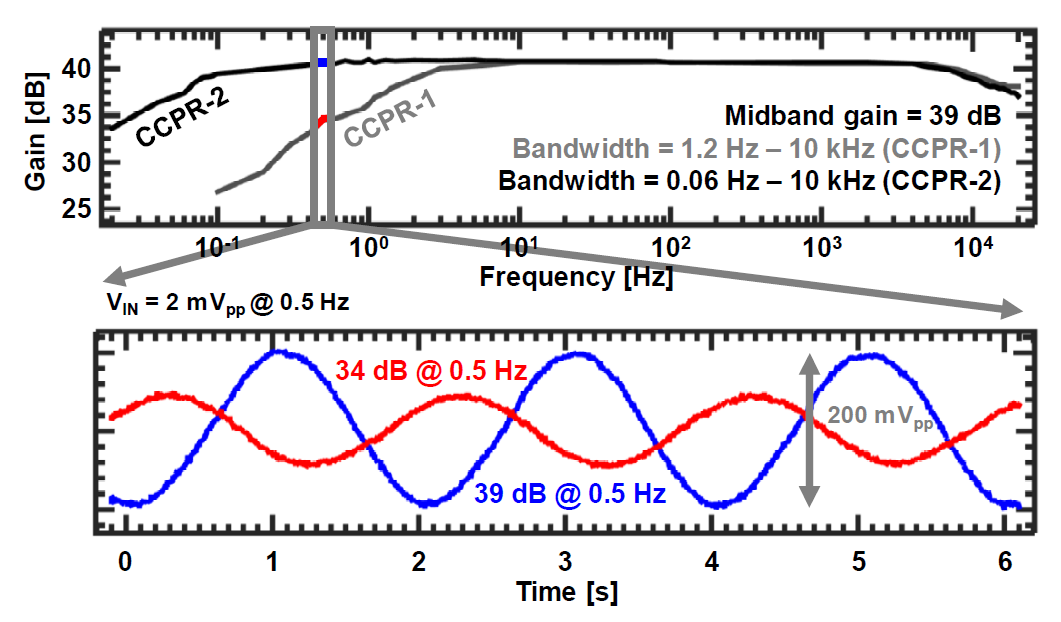Fig. 14. Output signals of the LNA when applying the input signals of 2 μVpp at 1 kHz (top), 2 kHz (middle), and 4 kHz (bottom).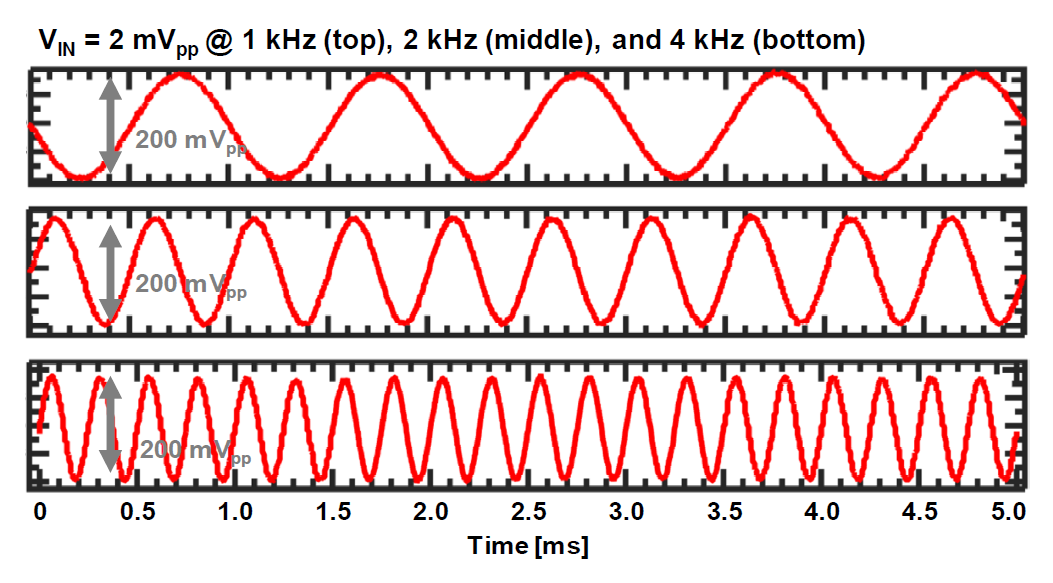The gain of the AFE changes from 61.9 dB to 73.9 dB by controlling the switches, $G_{1}$, $G_{2}$, and $G_{3}$, as shown in Fig. 8. To verify the operation of the AFE with the gain programmability, the output signals are measured by applying the sinusoidal input signal as shown in Fig. 15. In the bottom, the output signals of the AFE is plotted with varying the gain when the input signal of 100 μVpp at 1 kHz is applied using a low-distortion function generator. The input-referred noise performance of the AFE adopting four different kinds of pseudoresistors is presented in Fig. 16. The pink line represents the inputreferred noise of the AFE with the pseudoresistor based on diode-connected MOSFETs. The blue line is for the case of the voltage-controlled pseudoresistor. The red and green lines represent the cases of CCPR-1 and CCPR-2, respectively. Table 1 shows the comparison of the input-referred noise performance between the cases of four different kinds of pseudoresistors. The pseudoresistor formed by diode-connected MOSFETs undergoes the process variation. Therefore, there is a limit to achieve a high resistance. The voltage-controlled type can achieve a high resistance, but requires precise voltage regulation. The proposed current-controlled types of CCPR-1 and CCPR-2 achieve a high resistance with less calibration. For achieving a high resistance in CCPR-1 and CCPR-2, $I_{REF}$ is first copied from $M_{1}$ to $M_{5}$ with the ratio of 2000/1 in BG. Then, the current flowing through $M_{5}$ in BG is copied to $M_{R1}$ and $M_{R2}$ with the ratio of 500/1. The $M_{R3}$ and $M_{R4}$ maintain small $V_{GS}$, which allows a small current to flow through $M_{R5}$ and $M_{R6}$ to ensure a high resistance. The measured spot noise is about 30 nV/√Hz at 1 kHz. The integrated input-referred noise for all these cases is measured to be less than 3 μVrms.

Fig. 15. Frequency response of the neural recording front-end IC with different gains (top) and output signals using the input signal of 100 μVpp at 1 kHz (bottom).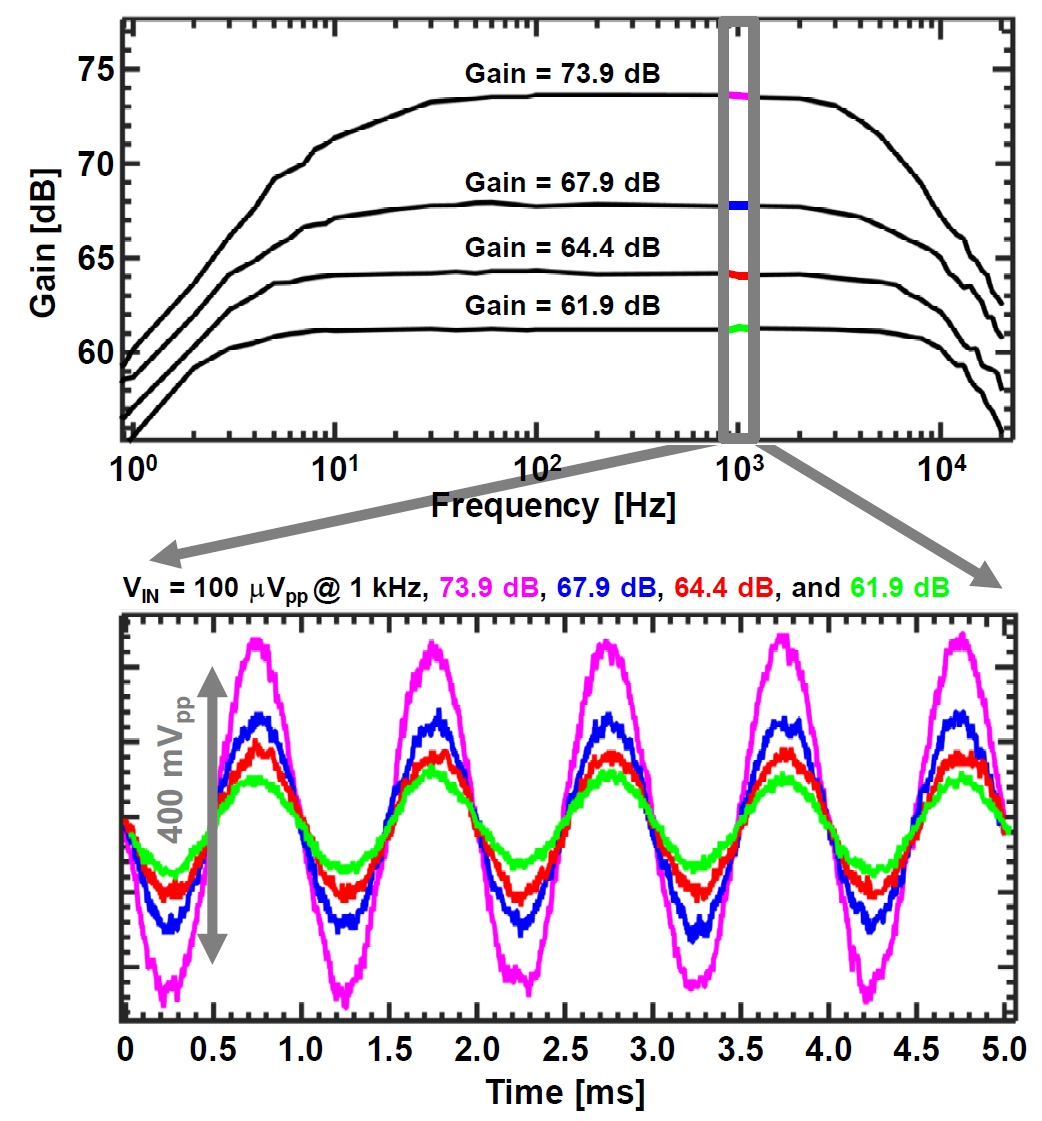Fig. 16. Measurement results of the input-referred noise with different kinds of pseudoresistors.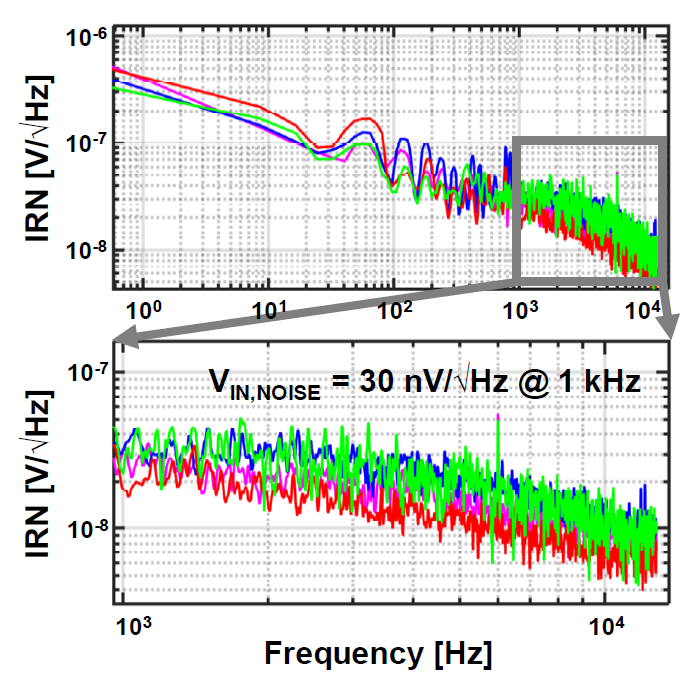Table 1. Comparison of the Input-Referred Noise Performance for Different Types of Pseudoresistors

 Pseudoresistor type Noise bandwidth Integrated IRN Diode-connected MOS 10 Hz - 10 kHz 2.50 μVrms Voltage-controlled MOS 1 Hz - 10 kHz 2.80 μVrms CCPR-1 1 Hz - 10 kHz 2.68 μVrms CCPR-2 0.5 Hz - 10 kHz 2.88 μVrms

Since the neural recording AFE should be designed to have low noise while consuming limited current, the tradeoff between the noise performance and current consumption is quantified by the noise-efficiency factor (NEF)[7,20], which is defined as

##### (1)
$\mathrm{NEF}=\mathrm{V}_{\mathrm{IRN}} \sqrt{\frac{2 I_{t o t}}{\pi \cdot U_{T} \cdot 4 k T \cdot \mathrm{BW}}}$

where $V_{IRN}$ is the integrated input-referred rms noise, $I_{tot}$ is the total current consumption, $U_{T}$ is the thermal voltage, $k$ is the Boltzmann constant, $T$ is the absolute temperature, and BW is the bandwidth of an amplifier.

Considering the decreasing supply voltage, the NEF can be modified to the power efficiency factor of NEF2VDD to quantify the tradeoff between the noise performance and power consumption,

##### (2)
$\mathrm{NEF}^{2} \mathrm{V}_{\mathrm{DD}}={V_{I R N}}^{2} \frac{2 P_{\mathrm{tot}}}{\pi \cdot U_{T} \cdot 4 k T \cdot \mathrm{BW}}$

where $P_{tot}$ is the total power consumption. The fabricated AFE achieves the NEF of 2.38 and the NEF2VDD of 5.67, respectively, with the power consumption of 4.6 μW/Channel. Table 2 summarizes the measured performances and compares the performances with other AFE designs. Fig. 17 shows the test PCB for the neural recording front-end IC (left) and the chip-on-board diagram (right). The die micrograph is shown in Fig. 18. The total size of IC is 2,200 μm × 3,840 μm with the single channel area of 82 μm × 1,260 μm.

Table 2. Measured Performance Summary and Comparison with Other Analog Front-End Designs

 C. M. Lopez, 2012 D. Han, 2013 C. M. Lopez, 2014 T. Lee, 2017 This work Process 0.35-μm 0.18-μm 0.18-μm 0.18-μm 0.18-μm Supply voltage 3.3-V 0.45-V 1.8-V 1.8-V 1-V Supply current 70 μA (AP range) 3.5 μA (LFP range) 1.62 μA 3.9 μA 1.6 μA 4.6 μA Gain 40-75.56 dB 52/55.56 dB 29.54-72 dB 40 dB 61.9/64.4/67.9/73.9 dB High-pass cutoff freq. 2.6/5.6/13.4/213/323/572 Hz 0.25 Hz 0.5/200/300/500 Hz 1 Hz 0.06 Hz Low-pass cutoff freq. 230 Hz/6.2 kHz 2.5 / 10 kHz 200 Hz/6 kHz 10 kHz 10 kHz Input-referred noise 2.3-2.9 μVrms 3.20 μVrms 3.20 μVrms 4.67 μVrms 2.88 μVrms NEF 5.1 1.57 3.08 2.27 2.38 NEF2VDD 85.8 1.12 17.13 9.28 5.67 Power/Ch 231 μW/Ch (AP range) 11.55 μW/Ch (LFP range) 0.73 μW/Ch 7.02 μW/Ch 2.88 μW/Ch 4.6 μW/Ch Area/Ch - - - 0.11 mm2/Ch 0.11 mm2/Ch Comparison blocks LNA/VGA/Buffer LNA/VGA Pixel amplifier/ PGA/Filter/Buffer LNA LNA/VGA/Buffer

Fig. 17. Test PCB for the neural recording front-end IC (left) and chip-on-board diagram (right).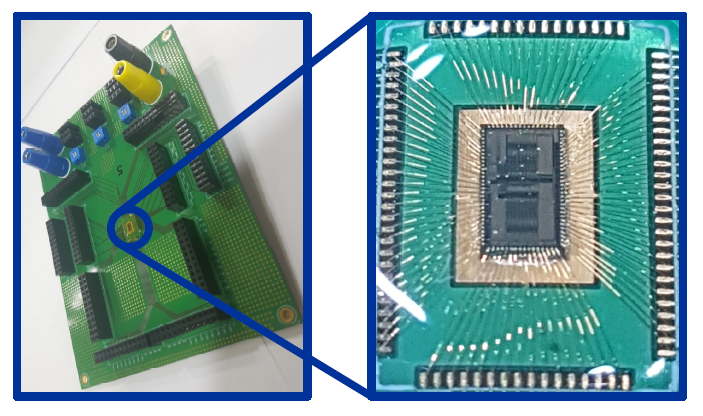## V. CONCLUSION

This paper demonstrates a 1-V 4.6 mW/Channel fully differential implantable neural recording front-end IC that occupies small area and consumes lower power. Thanks to the noise reduction and power saving techniques, the proposed chip achieves the recording performance required for successful implantable neural signal recording. The low-frequency signals are measured with minimal loss due to low enough fHPF which is realized using the current-controlled pseudoresistor. Comparing to other AFE designs, this IC achieves reasonable NEF and NEF2VDD. In addition, the narrow and long layout for each channel of the AFE is suitable for creating high-channel-density recording IC.

### ACKNOWLEDGMENTS

This research was supported by the Convergence Technology Development Program for Bionic Arm (2017M3C1B2085296) and the Brain Research Program (2017M3C7A1028859) through the National Research Foundation Korea (NRF) funded by Ministry of Science and ICT.

### REFERENCES

1
Christopher & Dana Reeve Foundation , 2013, Stats about paralysis, https://www.christopherreeve.org/living-with-paralysis/statsabout-paralysis2
Hochberg L. R., Serruya M. D., Friehs G. M., Mukand J. A., Saleh M., Caplan A. H., Branner A., Chen D., Penn R. D., Donoghue J. P., Jul. 2006, Neuronal ensemble control of prosthetic devices by a human with tetraplegia, Nature, Vol. 442, pp. 164-1713
Hochberg L. R., Bacher D., Jarosiewicz B., Masse N. Y., Simeral J. D., Vogel J., Haddadin S., Liu J., Cash S. S., Smagt P. V. D., Donoghue J. P., May 2012, Reach and grasp by people with tetraplegia using a neurally controlled robotic arm, Nature, Vol. 485, pp. 372-3754
Bouton C. E., Shaikhouni A., Annetta N. V., Bockbrader M. A., Friedenberg D. A., Nielson D. M., Sharma G., Sederberg P. B., Glenn B. C., Mysiw W. J., Morgan A. G., Deogaonkar M., Rezai A. R., May 2016, Restoring cortical control of functional movement in a human with quadriplegia, Nature, Vol. 533, pp. 247-2505
Guggenmos D. J., Azin M., Barbay S., Mahnken J. D., Dunham C., Mohseni P., Nudo R. J., Dec. 2013, Restoration of function after brain damage using a neural prosthesis, Proceedings of the National Academy of Sciences of the U. S. A. (PANS), Vol. 110, No. 52, pp. 21177-211826
Seese T. M., Harasaki H., Saidel G. M., Davies C. R., 1998, Characterization of tissue morphology, angiogenesis, and temperature in the adaptive response of muscle tissue to chronic heating, Lab. Invest., Vol. 78, No. 12, pp. 1553-15627
Harrison R. R., Charles C., Jun. 2003, A low-power lownoise CMOS amplifier for neural recording applications, IEEE Journal of Solid-State Circuits, Vol. 38, No. 6, pp. 958-9658
Huhta J. C., Webster J. G., Mar. 1973, 60-Hz interference in electrocardiography, IEEE Transactions on Biomedical Engineering, Vol. BME-20, No. 2, pp. 91-1019
Lee S., George A. K., Lee T., Chu J. U., Han S., Kim J. H., Je M., Lee J., Feb. 2018, A 110dB-CMRR 100dB-PSRR multi-channel neural-recording amplifier system using differentially regulated rejection ratio enhancement in 0.18mm CMOS, in Proc. IEEE Int. Solid-State Circuits Conf. (ISSCC) Dig. Tech. Papers, pp. 472-47310
Ng K. A., Xu Y. P., Oct. 2013, A compact, low input capacitance neural recording amplifier, IEEE Transactions on Biomedical Circuits and Systems, Vol. 7, No. 5, pp. 610-62011
Han D., Zheng Y., Rajkumar R., Dawe G. S., Je M., Dec. 2013, A 0.45 V 100-channel neural-recording IC with sub-$\mu$W/channel consumption in 0.18 $\mu$m CMOS, IEEE Transactions on Biomedical Circuits and Systems, Vol. 7, No. 6, pp. 735-74612
Ha S., Kim C., Chi Y. M., Akinin A., Maier C., Ueno A., Cauwenberghs G., May 2014, Integrated circuits and electrode interfaces for noninvasive physiological monitoring, IEEE Transactions on Biomedical Engineering, Vol. 61, No. 5, pp. 1522-153713
Park S. Y., Cho J., Lee K., Yoon E., Apr. 2018, Dynamic power reduction in scalable neural recording interface using spatiotemporal correlation and temporal sparsity of neural signals, IEEE Journal of Solid-State Circuits, Vol. 53, No. 4, pp. 1102-111414
Zou X., Liu L., Cheong J. H., Yao L., Li P., Cheng M. Y., Goh W. L., Rajkumar R., Dawe G. S., Cheng K. W., Je M., Oct. 2013, A 100-channel 1-mW implantable neural recording IC, IEEE Transactions on Circuits and Systems–I: Regular Papers, Vol. 60, No. 10, pp. 2584-259615
Liu L., Zou X., Goh W. L., Ramamoorthy R., Dawe G., Je M., Apr. 2012, 800 nW 43 nV/√Hz neural recording amplifier with enhanced noise efficiency factor, Electronics Letters, Vol. 48, No. 9, pp. 479-48016
Kim S. J., Liu L., Yao L., Goh W. L., Gao Y., Je M., Nov. 2014, A 0.5-V sub-$\mu$W/channel neural recording IC with delta-modulation-based spike detection, in Proc. IEEE Asian Solid-State Circuits Conference (A-SSCC), pp. 189-19217
Lee T., Jang D., Jung Y., Jeon H., Hong S., Han S., Chu J. U., Lee J., Je M., Oct. 2017, A neural recording amplifier based on adaptive SNR optimization technique for long-term implantation, in Proc. IEEE Biomedical Circuits and Systems Conference (BioCAS), pp. 1-418
Lopez C. M., Prodanov D., Braeken D., Gligorijevic I., Eberle W., Bartic C., Puers R., Gielen G., Apr. 2012, A multichannel integrated circuit for electrical recording of neural activity, with independent channel programmability, IEEE Transactions on Biomedical Circuits and Systems, Vol. 6, No. 2, pp. 101-11019
Lopez C. M., Andrei A., Mitra S., Welkenhuysen M., Eberle W., Bartic C., Puers R., Yazicioglu R. F., Gielen G. G. E., Jan. 2014, An implantable 455-active-electrode 52-channel CMOS neural probe, IEEE Journal of Solid-State Circuits, Vol. 49, No. 1, pp. 248-26120
Steyaert M. S. J., Sansen W. M. C., Zhongyuan C., Dec. 1987, A micropower low-noise monolithic instrumentation amplifier for medical purposes, IEEE Journal of Solid-State Circuits, Vol. SC-22, No. 6, pp. 1163-116821
Muller R., Gambini S., Rabaey J. M., Jan. 2012, A 0.013 mm2, 5 $\mu$W, DC-coupled neural signal acquisition IC with 0.5 V supply, IEEE Journal of Solid-State Circuits, Vol. 47, No. 1, pp. 232-243## Author

1994년 11월 1일생

2017년도 상명대학교 에너지그리드학과 졸업(공학사)

2017~현재 상명대학교 에너지그리드학과 재학(석사과정)

E-mail : 201311047@sangmyung.kr

1993년 2월 22일생

2017년도 상명대학교 에너지그리드학과 졸업(공학사)

2017~현재 상명대학교 에너지그리드학과 재학(석사과정)

E-mail : gnscjftls@naver.com